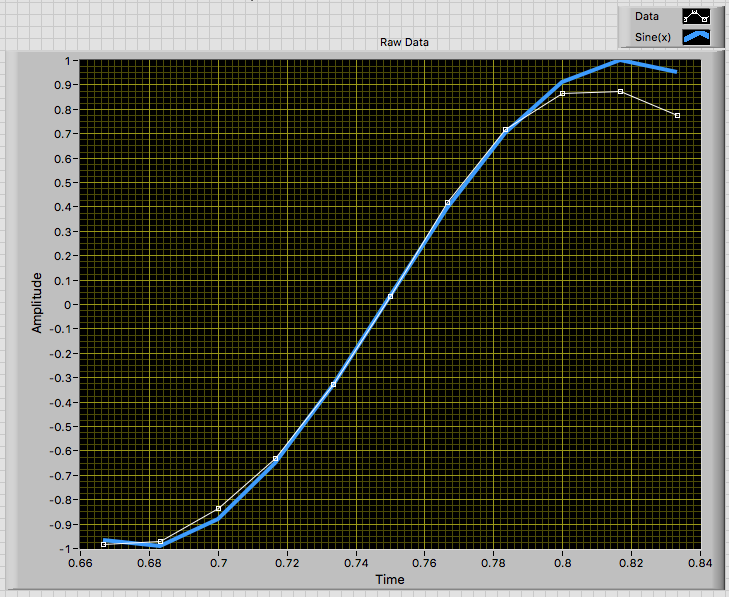# LabVIEW

cancel
Showing results for
Search instead for
Did you mean:

## Sine fit to evenly sampled data

I have evenly sampled data, where each data point corresponds to a point on a sine wave.  I need to detect the peak of the sine wave during a particular time window, and so I need to fit a sine wave to my data points.

For now, I have used a polynomial fit (attached LabVIEW 2013 code and screenshots), but this isn't good enough. Each data point I have should be on the sine-fit line.

Is there any way of doing this in LabVIEW?

Message 1 of 17
(2,432 Views)

## Re: Sine fit to evenly sampled data

Instead of looking into curve fitting functions, i recommend using a higher sample rate for acquisition. This results for more data to work on and there is a higher chance to have the maximum (peak as you call it)as a direkt sample.

Also note that measurement inaccuracies and rounding errors add up during analysis. Depending on the oversampling rate (sine frequency vs. sampling frequency), simply picking the maximum value could already be equally accurate.

Norbert

Norbert
----------------------------------------------------------------------------------------------------
CEO: What exactly is stopping us from doing this?
Expert: Geometry
Marketing Manager: Just ignore it.
Message 2 of 17
(2,406 Views)

## Re: Sine fit to evenly sampled data

Thanks for the suggestion, but I'm afraid this is not an option. I have years of data to analyse - this is just how the instrument has been working. I can't change the data in any way.

Message 3 of 17
(2,394 Views)

## Re: Sine fit to evenly sampled data

Then i recommend to discuss the maximum excepted error. Depending on the result, the recommended "just pick the maxmimum value" might still be valid.

If the basic signal is known to be a sine waveform, i'd rather use resample than interpolation...

Norbert

Norbert
----------------------------------------------------------------------------------------------------
CEO: What exactly is stopping us from doing this?
Expert: Geometry
Marketing Manager: Just ignore it.
Message 4 of 17
(2,391 Views)

## Re: Sine fit to evenly sampled data

Does your Sine change its frequency over time?

If not you just need two data points (x1,t1) and (x2,t2). Then you can calculate A and w from 2 equations with 2 variables

x1=A*sin(w*t1) and x2=A*sin(w*t2) with simple math. Your sine_max is A.

Message 5 of 17
(2,373 Views)

## Re: Sine fit to evenly sampled data

Do you know the frequency of the sinusoid, and the sampling frequency?  In that case, you can easily fit a sinusoid to the data, and use the phase information to tell you when the peak of each single sine wave occurs.

Can you attach an example data file (let us know the format so we can read it), tell us the sampling frequency, and one of us (such as I, unless someone beats me to it) will look at and suggest how to "find the peaks".

Bob Schor

Message 6 of 17
(2,366 Views)

## Re: Sine fit to evenly sampled data

@CtheR The sine wave doesn't change over time, but doesn't this give multiple answers for w, and hence A? E.g. take two points on my sine wave: x1=0.718, t1=0.7833 and x2=0.863, t2=0.8.  This gives, for example, w=0,4.45,8.79,13,... which leads to multiple possibilities for A.

Message 7 of 17
(2,355 Views)

## Re: Sine fit to evenly sampled data

The data you posted is not very sinusoidal. The image below shows a "manual" fit of a sine to your data. I adjusted the offset to match the zero crossing and then a multiplier to match the slope near the zero crossing. The plotted fit is sin(m*(x-b)) where be is the x value at the zero crossing and m is the multiplier.

Peak Detector.vi seems to work OK with your data. It finds the peak as x = 0.8096, which appears reasonable.

LynnMessage 8 of 17
(2,354 Views)

## Re: Sine fit to evenly sampled data

@Bob_Schor I don't know the frequency of the sinusoid. I just know the sampling frequency.  Example data is shown in the screenshot I attached.

Message 9 of 17
(2,351 Views)

## Re: Sine fit to evenly sampled data

Sorry, I didn't look at the pictures (pictures are almost never data, they are at best "pictures of data").  However, now that I have, I "see the problem" -- you may only have a fraction of a sinusoid represented by your data, which makes the problem "problematic".  A sinusoid is characterized by four numbers -- offset (DC value), amplitude, phase, and frequency.  If you have multiple cycles, you can estimate frequency, and if you know frequency, you can estimate the other three parameters easily.  If you have less than a cycle, the estimation problem becomes much more difficult.

I will repeat my request.  Can you attach a (complete) set of data?  I can't do any computations from a picture -- I need numbers (or a file whose format you describe to me so I can extract the numbers).

Bob Schor

Message 10 of 17
(2,342 Views)